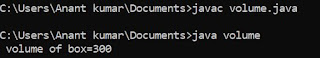## Sunday, 8 April 2018

### Program to maximum between two number in C

This is a program to find the maximum between two number in C.In this program firstly we have to input two number from the user and then we have to write condition for maximum between two number and there must be uses of operator for checking the conditions .So here we are going to also learn about operator use in C. The various operator of C are;
1.Arithmetic operator
2.Relational  "
3.Logical      "
4. Unary       "
5. Assignment "
6.Conditional "

/* maximum of two number*/
# include <stdio.h>
void main ()
{
int a,b;
clrscr ();
printf ("\n Enter the value of a:");
scanf ("%d",& a);
printf ("\n Enter the value of b:");
scanf ("%d",& b);
if(a>b)
printf ("a is maximum");
else
printf ("b is maximum");
getch();
}